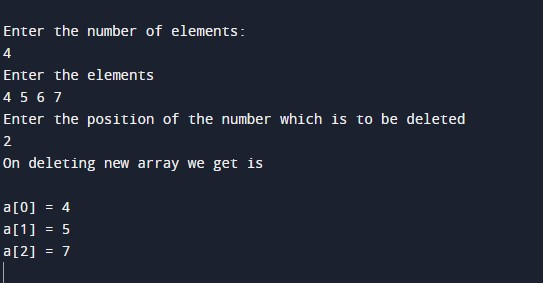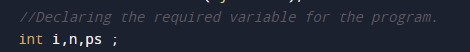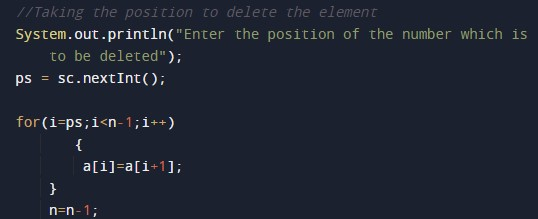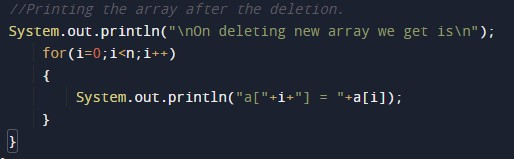# Java Program to Remove Element From Array

In this tutorial you will learn about the Java Program to Remove Element From Array and its application with practical example.

## Java Program to Remove the elements of an Array

In this tutorial, we will learn to create a Java program that will remove the elements of an Array using Java programming.

## Prerequisites

Before starting with this tutorial, we assume that you are the best aware of the following Java programming topics:

• Operators in Java Programming.
• Basic Input and Output function in Java Programming.
• Basic Java programming.
• For loop in Java programming.
• Arithmetic operations in Java Programming.

## Program description to find Remove the Elements of an Array.

In this program, we will first take the input array size and the elements from the user. Then we will take a position to remove an element of the array using a for-loop. At last, we will print the array to the user using the print function.

With the help of this program, we can Remove the Elements of an Array.

## Output:-In the above program, we have first initialized the required variable.• ps = it will hold the integer value of the input elements.
• i = it will hold the integer value.
• n = it will hold the integer value of the input.

Input size and the elements of the array from the user.Program Logic Code for the Remove of the elements of the array.Printing the array after deletion of the element.In this tutorial we have learn about the Java Program to Remove Element From Array and its application with practical example. I hope you will like this tutorial.